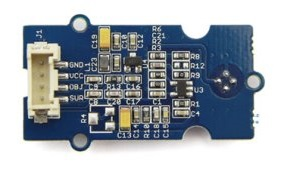UPM

The UPM API is a high level sensor library for IoT devices using MRAA. See examples here. Back to index page.
SparkFun sensor images provided under CC BY-NC-SA-3.0.

OTP538U Class

Module: otp538u

UPM module for the OTP538U IR temperature sensor
This module was tested with the Grove IR non-contact temperature sensor.
The sensor provides 2 analog outputs: one for the thermistor that measures the ambient temperature, and the other for the thermopile that measures the object temperature.
Much of the code depends on analyzing Seeed Studio* examples and the circuit design. As a result, there are several 'magic' numbers derived from their circuit design. These values are used by default.
The tables used came from the "538U VT Table__20_200(v1.3).pdf" and "538RT_table.pdf" datasheets.
These tables assume the object to be measured is 9 cm (3.54 inches) from the sensor.
This sensor will not work at 3.3v on the Edsion or the Galileo 2. It works fine on both systems at 5v. It will work at 3.3v on the Arduino 101 (tested via firmata subplatform on edison).Methods

`OTP538U`

(
• `pinA`
• `pinO`
• `aref`
)
Number

OTP538U constructor

Parameters:

• `pinA` Number

Analog pin to use for the ambient temperature

• `pinO` Number

Analog pin to use for the object temperature

• `aref` Number

Analog reference voltage; default is 5.0 V

Number:

`ambientTemperature`

() Number

Gets the ambient temperature in Celsius

Returns:

Number:

Ambient temperature

`objectTemperature`

() Number

Gets the object temperature in Celsius

Returns:

Number:

Object temperature

`setVoltageOffset`

(
• `vOffset`
)

Sets the offset voltage
The Seeed Studio wiki gives an example of calibrating the sensor and calculating the offset voltage to apply. Currently, the default value is set, but you can use the function to set one of your own.

Parameters:

• `vOffset` Number

Desired offset voltage

`setOutputResistence`

(
• `outResistance`
)

Sets the output resistance value
The Seeed Studio wiki example uses a value of 2,000,000 in one of the equations used to calculate voltage. The value is the resistance of a resistor they use in the output stage of their SIG2 output. This was 'decoded' by looking at the EAGLE* files containing their schematics for this device.

Parameters:

• `outResistance` Number

Value of the output resistor; default is 2M Ohm

`setVRef`

(
• `vref`
)

Sets the reference voltage of the internal Seeed Studio voltage regulator on the sensor board.
The Seeed Studio wiki example uses a value of 2.5 in one of the equations used to calculate the resistance of the ambient thermistor. The value is the voltage of an internal voltage regulator used on the sensor board. This was 'decoded' by looking at the EAGLE files containing their schematics for this device.

Parameters:

• `vref` Number

Reference voltage of the internal sensor; default is 2.5

`setDebug`

(
• `enable`
)

Enable debugging output.

Parameters:

• `enable` Boolean

true to enable some debug output, false otherwise